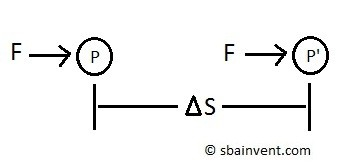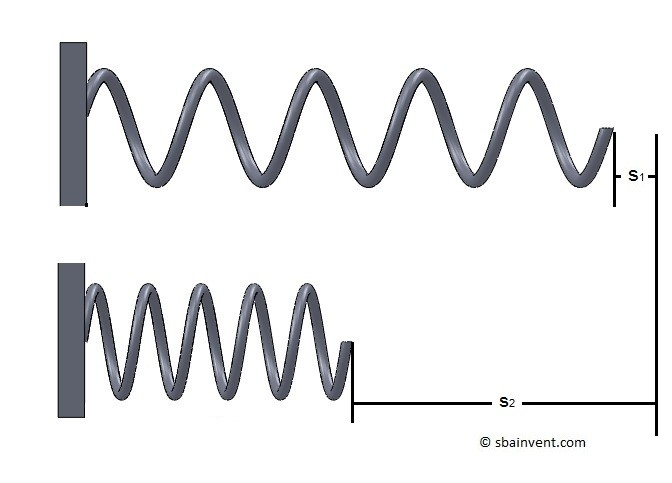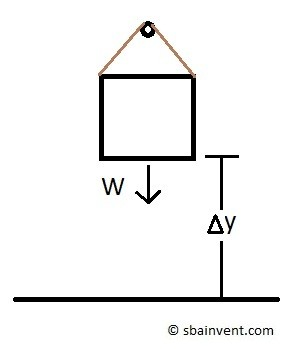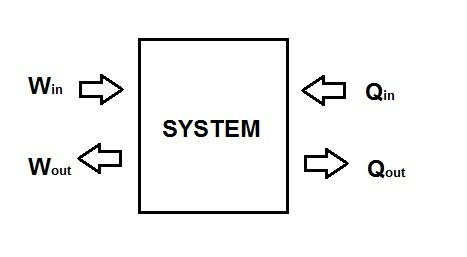# Energy Transfer

Thermodynamics studies the changes in the total energy of a system. This is directly related to energy transfer from one system to another system, or a system to its surroundings. When dealing with a closed system energy transfer occurs across the boundaries of the system. There are two types of energy transfer that can occur in a closed system, and they are energy transferred by heat and energy transferred by work. Energy transfer only occurs across the boundaries of the system, or a fluid flowing through the system. Once the energy has been transferred it becomes part of the internal energy of the system or the environment.

##### Energy Transferred by Heat

An example of energy transferred by heat is when you leave a hot cup of coffee out in room temperature for too long it will cool down to room temperature. From this example, a simple definition for energy transferred by heat can be derived. Simply if energy is being transferred by heat then there has to be a temperature difference between two systems, or a system and its environment. Due to this temperature difference, energy will be exchanged until the two systems are in thermal equilibrium. Once the two systems are in thermal equilibrium (at the same temperature) then energy can no longer be transferred by heat, and if energy is still crossing the boundary of the system then the energy transfer must be due to work. During a thermodynamic process if no heat crosses the boundary of the system then it can be considered an adiabatic process. Heat transfer can be represented in per unit mass form by using the following equation.

(Eq 1)  $q=\frac{Q}{m}$

There are three distinct forms of heat transfer. They are conduction, convection, and radiation. Conduction occurs when two particles come in contact with each other and the more energetic particle transfers energy to the least energetic particle. Convection is very similar to conduction, except the heat exchange occurs when a fluid flows over a solid. Finally, heat transfer due to radiation occurs due to the emission of electromagnetic waves from the object. A prime example of heat transfer due to radiation is the heat that comes from the sun.

##### Energy Transferred by Work

Similar to heat energy, energy due to work is measured by the energy the crosses the boundaries of the system. So if you know that that there is energy crossing the boundaries of the system, and it is not heat, then that means it must be work.

So what exactly is work? Work is the energy transferred as a force acts through a certain distance. This can be simply defined as the equation below.

(Eq 2)  $W=Fs$

$F$ = Force

$s$ = distance traveledEquation 2 is the simplest equation to express mechanical work. However, there are more specific equations that can represent work. For example the work of a rotating shaft that is rotating at a constant speed is expressed by equation 3.

(Eq 3)  $W_{sh}=2πnT$

$n$ = number of rotations

$T$ = Torque

In equation 3, n represents the number of revolutions, while T represents the torque applied to the shaft to cause it to rotate. Next, you can modify equation 3 to find the power required to rotate the shaft; refer to equation 4.

(Eq 4)  $\dot{W}_{sh}=ωT$

$ω$ = Angular Velocity

In equation 5, ω represents that angular velocity of the shaft as it rotates. Now if you compare equation 4 to equation 3 you should notice that equation 3 is simply work over a unit time. This means if you want to know how much power is required to do a certain amount of work in a specific amount of time all you would have to do is divide work by time to obtain the required power.

You can also calculate the required work to compress or stretch a spring by using the following equation.

(Eq 5)  $W_{spring}=\frac{1}{2}k\left(x_2^2-x_1^2\right)$

$k$ = Spring constantEquation 5 can also be applied to solid shafts that are under a normal force once you calculate that shafts stiffness. To learn more about how to calculate the stiffness of a shaft refer to the strength of materials section.

Finally, a change in energy due to work can be related to lifting a body up a certain height; refer to equation 6.

(Eq 6)  $W_{pe} =mgz$

$m$ = mass

$g$ = gravitational constant

$z$ = heightFrom equation 3-6 you may have noticed that these equations represent a potential or a kinetic energy equation. So from that, if you can calculate the potential or kinetic energy due to some mechanical change, then you can effectively calculate the change in energy due to mechanical work.

In addition to mechanical work, you can change a systems total energy through electrical work. To do this you multiply electrical power by some unit time; refer to equation 8. Equation 7 represents electrical power.

(Eq 7)   $\dot{W}_e =VI$

$V$ = Voltage

$I$ = Current

(Eq 8)  $W_e=VIΔt$

$t$ = time

From equations 7 and 8, V represents the electrical voltage and I represents the electrical current.

##### Similarities of Heat and Work

Both heat and work have some similarities in the way they behave that you will have to consider. First both heat and work are directional quantities. This means that both of them have a certain magnitude and direction in relation to whether the energy is entering or leaving the system. Refer to the figure below.Second, both heat and work are only recognized when energy crosses the systems boundaries. Third, building upon the second statement, systems only contain energy not work or heat. Fourth, both heat and work are associated with a process as the system follows a path from one state towards another state; refer to the figure below.